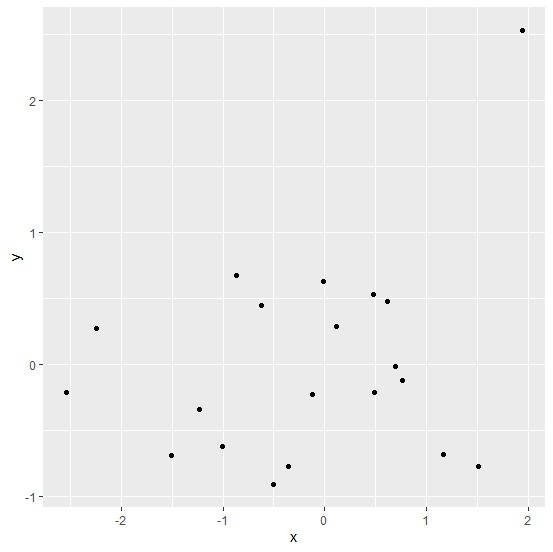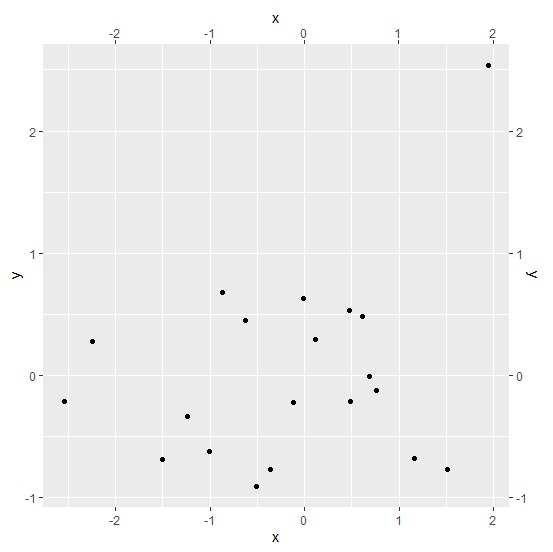# How to display tick marks on upper as well as right side of the plot using ggplot2 in R?

R ProgrammingServer Side ProgrammingProgramming

To display tick marks on upper as well as right side of the plot, we can create duplicate axes for X as well Y by using scale_x_continuous and scale_y_continuous functions. The argument that will help us in this case is sec.axis and we need to set it to dup_axis as scale_x_continuous(sec.axis=dup_axis()) and scale_y_continuous(sec.axis=dup_axis()). Check out the below example to understand how it can be done.

## Example

Consider the below data frame −

Live Demo

x<-rnorm(20)
y<-rnorm(20)
df<-data.frame(x,y)
df

## Output

       x             y
1   0.11204135   0.29084737
2   0.61199138   0.48121914
3   0.48760949  -0.21450790
4  -0.87200194   0.67532122
5  -2.24557725   0.27402959
6   0.76593441  -0.12197485
7   1.94506052   2.53583915
8  -0.35843587  -0.77521246
9  -1.50236224  -0.68711682
10 -0.11789693  -0.22534300
11  1.51156266  -0.77355032
12 -0.50594024  -0.91056427
13  0.69102879  -0.01354832
14 -0.62664213   0.44946662
15 -1.00403558  -0.62376588
16 -0.01299667   0.62897816
17 -1.23493618  -0.33727209
18  1.16264196  -0.68264496
19 -2.53843314  -0.21478113
20  0.47694554   0.52879296

## Example

library(ggplot2)
ggplot(df,aes(x,y))+geom_point()

## OutputCreating scatterplot between x and y with axes on upper and right side of the plot −

## Example

ggplot(df,aes(x,y))+geom_point()+scale_x_continuous(sec.axis=dup_axis())+scale_y_continuous(sec.axis=dup_axis())

## Output